One of the major classification algorithm we would be learning in this topic is called Naive bayes, this algorithm is based on fundamentals of probability, if you recall our previous topic i.e K-NN it was simple neighbourhood based technique, while naive bayes that we are going to learn today comes from the basics of probability. Before diving into our algorithm let’s first understand some fundamentals of probability.

## Conditional probability

What conditional probability means is P(A | B), where A and B are any random variable, and “|” indicates “given” or “conditioned on”. So if we read out this formula it says, probability of A having any random value given the random value of B.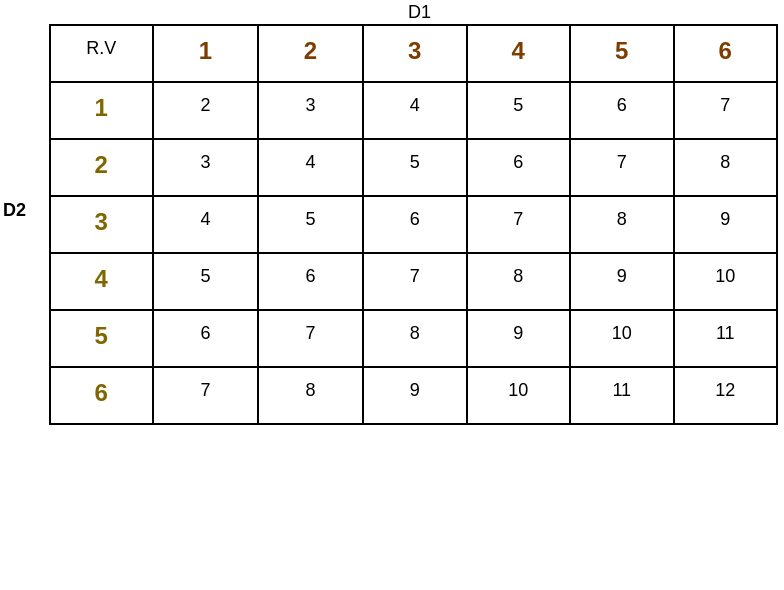We have played roll-a-dice game in our childhood and also saw some wicked smart hero rolling a dice in casino and taking all money with him right? Now imagine you are asked to throw two dice,  D1 be the random variable or we can say a random value that we got on first die and D2 be the random variable that we got on second die.

So, total out come that you will have for first die would be 6, and total outcome that you will have on the second die would be 6 as well, hence the total possible out come for both the die thrown together would be 6×6 i.e 36. In the descriptive image it is given, the sum of the value of the dice. So each cell is the sum of  D1 and D2.

What is the probability that D1+D2 <=4 ?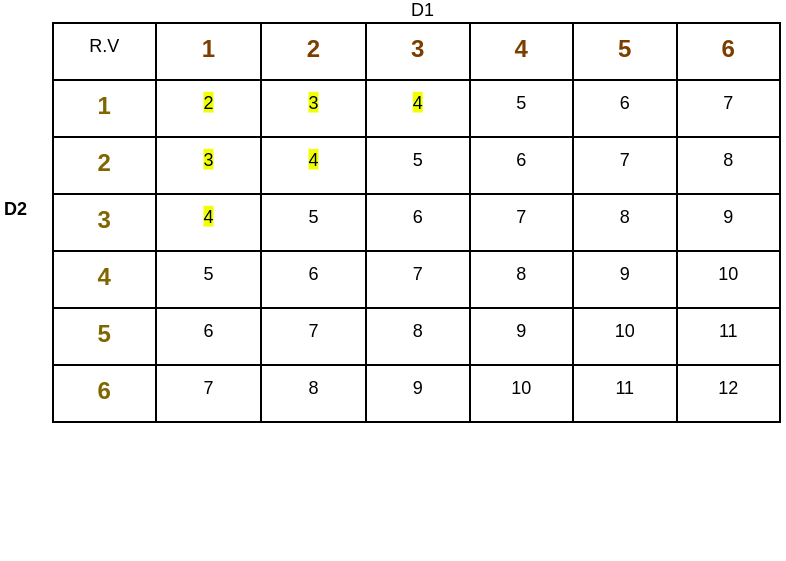Above question simply means, we have 36 possibilities, and out of this 36 possibilities how many outcomes is the sum of D1 and D2 less than or equal to 4. So the 6 yellow colored values are the out comes whose sum is less than or equal to 4. Hence the P(D1+D2<=4)=6/36

What is the probability that D1=2 given that D1+D2 <=4 ?Above question means, P(D2=2 | D1+D2 <= 4) Let’s solve this question, So there are 6 possibilities where D1+D2 <=4 and out of this 6 possibilities how many of them have D2 = 2. There are 2 blue colored who satisfy our question. So P(D2=2 | D1+D2 <= 4) = 2/6.

Let, unravel the formula we have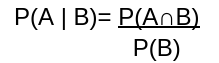What  P(A∩B) means, event A has happened as well as event B has happened. So let’s assume event A is D2=2 and event B is D1+D2 <=4  So it means there are only 2 possibilities out of 36 that satisfy P(A∩B) as shown in the image, so 3/36 is the numerator probability and probability of event b happening is 6/36 (we got it from above example)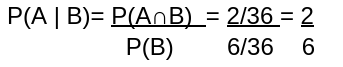To know more about Conditional probability, wikipedia has this amazing content that you can refer  https://en.wikipedia.org/wiki/Conditional_probability

## Bayes' theorem

Bayes’ theorem is simple and very useful theorem and it is from early 1700’s age

it is been named after Thomas Bayes. Bayes from Naive bayes comes from bayes’ theorem. So the theorem statesBefore jumping into the proof let’s understand some terminology in Bayes’ theorem.

P(A | B) is called posterior.

P(B | A) is called likihood.

P(A) is called prior.

P(B) is called evidence.

In alot of literature P(A∩B) is also written as P(A,B), both are same.

Proof:## Naive Bayes theorem

As we all know bayes term in naive bayes comes from bayesian theorem and naive term intuitively means unsophisticated/ simplistic. Now let’s go by the flow and understand its theorem.

Imagine we have dataset X and it has ‘n’ features and let’s assume we have ‘k’ classes and each classes is represented as ck if k=2 i will be considered as binary classification. Now we have x right, so we want to find out what is the probability given x what is its class label:- P(Ck | x) reading it aloud it states, probability of class label given datapoint x, and ‘x’ is x1,x2,x3….xn as it has ‘n’ features. So using bayes theorem we can write it as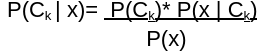Now let’s unravel numerator and denominator for better understanding, so intuitively what we will be doing is, highest probability value would be our class label, so in a nutshell denominator would be same for every possible k right, it won’t be a factor of having highest probability score. So let’s ignore the denominator and focus on numerator. So what we have learned uptill now and conditional probability we can say P(Ck)*P(x |Ck) = P(Ck ∩ x) often written as P(Ck ,x) this distrubution is called joint probability model.

There is something called the chain rule for conditional probability.

As we know (A ⋂ B) = (B ⋂ A) in same way

P(Ck ,x1,x2…xn) = P(x1,x2…xn,Ck)

Now if we think x1 as A and all of this term(x2,x3,…xn,Ck)as our B.

So probability of A ⋂ B is P(A | B)*P(B) by conditional probability right!!!

P(x1,x2…xn,Ck)=P(x1 | x2…xn,Ck) * P(x2…xn,Ck) ….. (1)

Now we will keep our first term as is and focus on 2nd term which is

P(x2,x3….xn,Ck)

Now if we use x2 as A and all of this term(x3,x4,…xn,Ck)as our B.

What we get is P(x2,x3…xn,Ck)=P(x2 | x3,x4…xn,Ck) * P(x3…xn,Ck)

After replacing it in equation 1 we get

P(x1,x2…xn,Ck)=P(x1 | x2…xn,Ck) * P(x2 | x3,x4…xn,Ck) * P(x3…xn,Ck)

So when we follow this same step till the last term we get is

P(x1,…xn,Ck)=P(x1 | x2…xn,Ck)*P(x2 | x3,…xn,Ck)*P(x3…xn,Ck)…P(xn-1 | xn,,ck)*P(xn | ck)*P(ck)

What naive bayes says is let’s make a conditional independence assumption.

Let’s take a complex example, if P(A |B,C)= P(A | C)

It means A is conditional independent of B

So P(xi | xi+1 , x1+2,…xn, ck)= P(xi | ck) it means xi  is independent of xi+1 , x1+2,…xn given class label ck

Hence, expanding and re-ordering it we will get

P(ck | x1, x2 …,xn) = P(ck) * P(x1 | ck) * P(x2 | ck)…..

To get a more better understanding you can refer

https://en.wikipedia.org/wiki/Naive_Bayes_classifier

## Lapace smoothing for text features

Imagine we have text dataset consisting of ‘m’ words in it, so in our training phase we will be needed probability of class label to know which class it belong and also probability of words to determine the importance and interpretibility. It is such as

P(w1 | y=0) & P(w1 | y=1)

P(w2 | y=0) & P(w2 | y=1)

..

P(wm | y=0) & P(wm | y=1)

After our training phase is over, in our test phase we are given a test query

Test_query=(w1,w2,w3,w’): w1,w2,w3 exist in my corpus(training data) and we have their probabilities. But w’ is not present, it is completely new word that we saw in test query. so now the challange is how do we get   P(w’|y=1) or P(w’ | y=0) . We can’t drop it, dropping w’ is equivalent to saying P(w’ | y=1)=1 and P(w’ |y=0)=1 which is incorrect.

So Laplace smoothing comes to our help(most of the people get’s confuse cosndiering it as lapkcian smoothing whic is ued for image processing) also known as additive smoothing.

1.  Get no. of times w’ occurs in training data where y=1 i.e 0 and no. of point where y=1 , and divide them :- No.points w’ occurs & y=1 / No. of points where y=1
2. Adding α to numerator and αk to the denominator, typically α is taken as 1 and k is distinct value w’ can take, i.e if there are 2 class label w’ can take 2 values. In our case its 0,1 so distinct values w’ can take is 2. So formula for laplace smoothing we get is No.points w’ occurs & y=1 + α  / No. of points where y=1 + αk

## Getting hands-on

We will be using Weka, an open source software to get hands-on experience of Naive Bayes. Before diving into code let’s understand what is weka. Weka is a collection of machine learning algorithms for data mining tasks. It contains tools for data preparation, classification, regression, clustering. Math over here would be taken care by WEKA. This is where you will learn more about it. https://www.cs.waikato.ac.nz/ml/weka/

Let’s import some required library, loading our data set into memory and separate variable and class that we want to predict.

				
import weka.core.converters.ConverterUtils
import weka.classifiers.bayes.NaiveBayes



Initially we will load data from the github respository, so that we may not need to download the data.

				
val data = weka.core.converters.ConverterUtils.DataSource("https://raw.githubusercontent.com/nmltd/s2-data-sets/main/iris.arff").getDataSet()




Let’s set label column i.e class to the last attribute of data

				
if (data.classIndex() == -1)
data.setClassIndex(data.numAttributes() - 1)



Weka have amazing filters in modules of theirs, filters are used for custom filtering of data, so we will be using stratified filter on our dataset to randomly fold the data and also lets split the data in 5 folds and select 1st fold for our usecase becasuse we are not using test data for this topic, we are dividing our whole data that we have to train and test data.

				
val options = arrayOf("-N", "5","-F","1")
filter.setOptions(options)
filter.setInputFormat(data)
filter.setInvertSelection(false)



So  let’s apply the filter that we created to the test data.

				
val test = weka.filters.Filter.useFilter(data, filter)
filter.setInvertSelection(true);     // invert the selection to get other data
val train = weka.filters.Filter.useFilter(data, filter)



Now its time to load and use our naive bayes classifer.

				
val cls = weka.classifiers.bayes.NaiveBayes();
cls.buildClassifier(train)



Finally let’s evaluate the classifer and see some statistics.

				
val eval = weka.classifiers.Evaluation(train)
eval.evaluateModel(cls, test)
print(eval.toSummaryString("\nResults\n======\n", false))We now conclude the topic, “Naive Bayes”.

Thank you for spending time on this course! Hope you learned a lot.